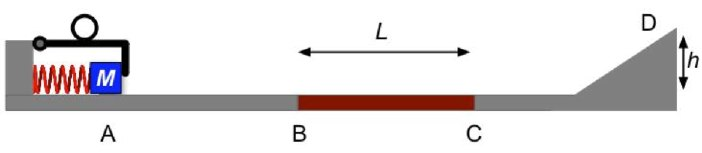Teaching Physics with the Physics Suite Edward F. Redish Problems Sorted by Type | Problems Sorted by Subject | Problems Sorted by Chapter in UP

Launching a block up a ramp

The apparatus shown in the figure below uses a spring to launch a small block along a track. A latch holds the block of mass M in place against the compressed spring (spring constant k) as shown. When the ring is raised the block is launched along the track. The parts of the track from A to B and from C to D can be considered frictionless for this problem. The part from B to C has significant friction (coefficient of sliding friction, µ).When the block is launched, it gets through the frictional part, rises up the ramp to the point D, a height h above the straight part of the ramp. It then slides back down. On its return, it gets to the point B and stops. For the items below choose the symbol that best completes the sentence and put it in the box on the right of the question: Use one of the following symbols: greater than (>); less than (<); equals (=), positive (+), negative (-), zero (0). If none complete the sentence correctly, put N.

(a) The potential energy stored in the spring just before the mass is released is ____ the kinetic energy of the mass as it is reaches the point B for the first time.

(b) The potential energy stored in the spring just before the mass is released is ____ the gravitational potential energy of the mass as it is reaches the point D. (Take the zero of gravitational PE at the level of the straight part of the track.)

(c) In the overall motion, the magnitude of the total work done by the frictional force is _____ the magnitude of the total work done by the spring force.

(d) The total work done by the gravitational force throughout the whole motion is _____.

(e) The total work done by the friction force throughout the whole motion is _____.